### IMO Shortlist 1962 problem 3

Kvaliteta:
Avg: 3,0
Težina:
Avg: 6,0
Consider the cube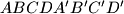$ABCDA'B'C'D'$ ($ABCD$ and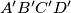$A'B'C'D'$ are the upper and lower bases, repsectively, and edges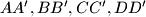$AA', BB', CC', DD'$ are parallel). The point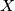$X$ moves at a constant speed along the perimeter of the square$ABCD$ in the direction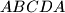$ABCDA$, and the point$Y$ moves at the same rate along the perimiter of the square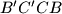$B'C'CB$ in the direction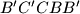$B'C'CBB'$. Points$X$ and$Y$ begin their motion at the same instant from the starting positions$A$ and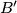$B'$, respectively. Determine and draw the locus of the midpionts of the segments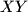$XY$.
Izvor: Međunarodna matematička olimpijada, shortlist 1962